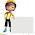## Search This Blog

### Difference Between Electrical Energy and Electrical Power

As we know that the electrical energy and electrical power both are the two major terms associated with electrical and electronics systems. The main fundamental difference between electrical energy and electrical power is the electrical energy represents the amount of work done that causes an electric current to flow through a circuit. As the against or electrical power is defined as the rate at which work is done is an electrical circuit. and the rate is specified according to the unit time. Now let us discuss the basic key difference between electrical energy and electrical power to better understand this topic.

Definition of electrical energy:

Electrical energy is defined as the overall work done in an electrical circuit. The energy is specified as the amount of work done to move an object and the electrical circuit. Thus the work done on the electric charge in order to cause movement is known as the electrical energy.

Definition of electrical power:

Electrical power is defined as the rate at which it is done on an electrical system. As we have discussed recently that doing work generates energy. Thus the power can be given as the rate of consumption of electrical energy.

## The main key difference between electrical energy and electrical power:

• The SI unit of the electrical energy is joules and electrical power is measured in watts.
• Energy is denoted by E while in P is used to denote electrical power.
• Electrical energy defines the energy granted due to the movement of charge carriers in a conductor while electrical power specifies the rate of consumption of electrical energy by a device.
• The produce of power and time give the value of energy however power is the produce of potential difference existing across 2 ends of a conductor and the current flowing through it.
• The electrical energy is defined as the E = VI.t while in Electrical power is defined as the P = V*I.
• Electrical energy is the energy generated as the result of the potential difference in a circuit which causes current to flow through it while the electrical power is the rate of the amount of electrical energy transferred.
As we know that the electrical energy and electrical power both are the two major terms associated with electrical and electronics systems. The main fundamental difference between electrical energy and electrical power is the electrical energy represents the amount of work done that causes an electric current to flow through a circuit. As the against or electrical power is defined as the rate at which work is done is an electrical circuit. and the rate is specified according to the unit time. Now let us discuss the basic key difference between electrical energy and electrical power to better understand this topic.

Definition of electrical energy:

Electrical energy is defined as the overall work done in an electrical circuit. The energy is specified as the amount of work done to move an object and the electrical circuit. Thus the work done on the electric charge in order to cause movement is known as the electrical energy.

Definition of electrical power:

Electrical power is defined as the rate at which it is done on an electrical system. As we have discussed recently that doing work generates energy. Thus the power can be given as the rate of consumption of electrical energy.

## The main key difference between electrical energy and electrical power:

• The SI unit of the electrical energy is joules and electrical power is measured in watts.
• Energy is denoted by E while in P is used to denote electrical power.
• Electrical energy defines the energy granted due to the movement of charge carriers in a conductor while electrical power specifies the rate of consumption of electrical energy by a device.
• The produce of power and time give the value of energy however power is the produce of potential difference existing across 2 ends of a conductor and the current flowing through it.
• The electrical energy is defined as the E = VI.t while in Electrical power is defined as the P = V*I.
• Electrical energy is the energy generated as the result of the potential difference in a circuit which causes current to flow through it while the electrical power is the rate of the amount of electrical energy transferred.

1.The content you've posted here is fantastic because it provides some excellent information that will be quite beneficial to me. Thank you for sharing about testing and tagging adelaide. Keep up the good work.

2.Acknowledges for paper such a beneficial composition, I stumbled beside your blog besides decipher a limited announce. I want your technique of inscription. electricity

3.It's so good and so awesome. I am just amazed. I hope that you continue to do your work like this in the future also.
This is an awesome motivating article.I am practically satisfied with your great work.You put truly extremely supportive data. Keep it up. electricity

4.Great! your blog is very interesting and informative because this blog contains meaningful content. If your are looking for these ev charging point operators in india. Our company provides the best services in India

5.the electricity being charged at a higher rate than on the rates for the Economy 7 duty, this may as yet be the most savvy choice in this sort of situation.capacitores de cerâmica de alta tensão